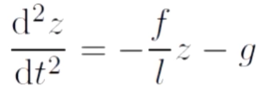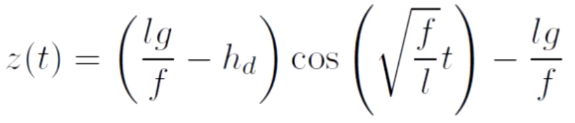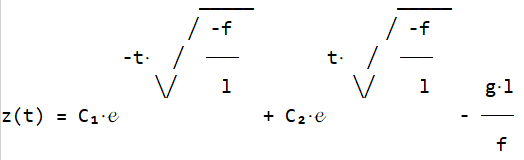2020-07-29 08:09

# Python sympy解一个二阶微分方程，输出通解怎么把常量的形式表示出来``````from sympy import *
init_printing(use_unicode=True)
z = Function('z')
t = symbols('t')
f = symbols('f')
l = symbols('l')
g = symbols('g')
dsolve(Eq(z(t).diff(t, t) + f / l * z(t), -g), z(t))
``````Python控制台输出的是这个鬼东西，我一看他就没把f，l，g当做常量去解。

• 点赞
• 写回答
• 关注问题
• 收藏
• 邀请回答